Next: 6.4.3.2 Multiple Shooting Up: 6.4.3 Shooting Method Previous: Single Shooting

### 6.4.3.1 Shooting with Reduced Superposition

In case of single shooting the ODE system has to be integrated N + Ns times: N times to determine the fundamental solution matrix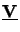(h) at the opposite boundary point z = h and another Ns times to determine the BVP solutions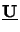(z) subject to the Ns BCs since the fundamental solution matrix is not stored at each mesh point to keep the memory demands small. However, the N BCs are equally separated at the two ending points z = 0 and z = h. It suffices to integrate the ODE system only N/2 times to relate both boundaries as will be shown. Since N/2 BCs are given at one point we can express the solution u(z) of our separated BVP by a reduced superposition like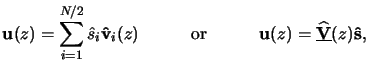(6.41)

whereby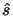is a N/2-dimensional coefficient vector and the N x N/2-dimensional matrix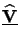(z) carries the N/2 linearly independent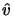i(z) spanning the vector spaceN x N/2. Now the initial values cannot be chosen arbitrarily. On the contrary, they have to fulfill the N/2 BCs at one ending point. Since we have homogeneous BCs at the lower interface at z = h we determine(h) at this point, i.e., we search for a matrix(h) that obeys (cf. (6.51))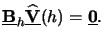(6.42)

For that we calculate the QR-factorization [202, pp. 223-236] of the complex-conjugate transposed or Hermitian of the boundary matrix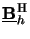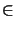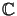N x N/2 (cf. Table 6.1),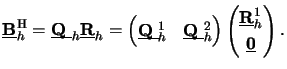(6.43)

Here,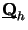N x N is an orthogonal matrix, whereasN x N/2 is upper triangular. The two sub-matrices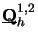are of dimension N x N/2, and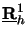and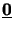are N/2 x N/2-dimensional. Since the inverse of an orthogonal matrix simply equals its Hermitian we obtain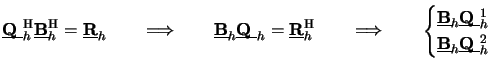(6.44)

The last equation is the required BC (6.63), and we thus have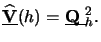(6.45)

The missing steps equal those performed in simple shooting (cf. Table 6.2). The big advantage stems from the fact that we halved the number of required integrations to obtain the linearly independent solution matrix at the opposite interval boundary (cp. (6.56) with (6.62)). The reduced superposition algorithm is summarized in Table 6.3. Numerical problems are moderated since the size of the linear equations system is also halved--compare Step 3 of Table 6.3 with Step 2 of Table 6.2--and can thus be inverted in a more stable way. As a final remark note that the ODE is now integrated in opposite'' direction as before, i.e., from z = h to z = 0 (cf. Figure 6.4).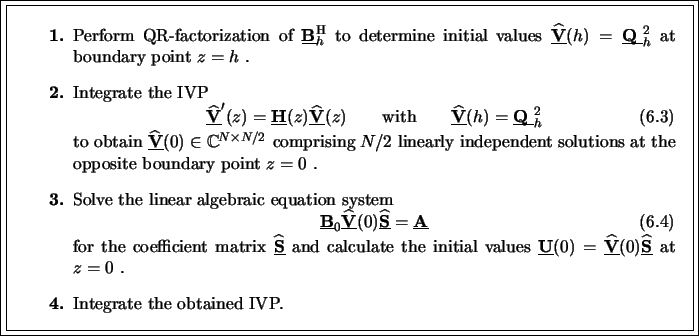Next: 6.4.3.2 Multiple Shooting Up: 6.4.3 Shooting Method Previous: Single Shooting
Heinrich Kirchauer, Institute for Microelectronics, TU Vienna
1998-04-17